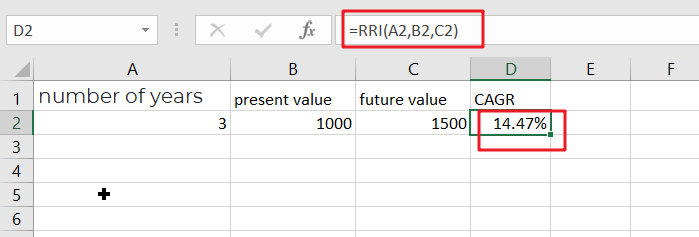# How To Use Excel RRI Function

This post will guide you how to use Excel RRI function with syntax and examples in Microsoft excel.

## Description

The Excel RRI function Returns an equivalent interest rate for the growth of an investment. So you can use the RRI function to get the the growth of an investment based on present and future value of the investment.

The RRI function is a build-in function in Microsoft Excel and it is categorized as a Financial Function.

The RRI function is available in Excel 2016, Excel 2013, Excel 2010, Excel 2007, Excel 2011 for Mac.

## Syntax

The syntax of the RRI function is as below:

`= RRI (nper,pv,fv)`

Where the PV function arguments are:

• nPer -This is a required argument. The total number of periods for the investment.
• pv – This is required argument. The present value is the future value of the investment
• fv – This is required argument. The future value is the future value of the investment.

Note:

• If argument values are not valid, the RRI function will return the #NUM! error.
• If argument values are not using valid date types, the RRI function will return the #VALUE! Error.

## Excel RRI Function Examples

The below examples will show you how to use Excel RRI Function to an equivalent interest rate for the growth of an investment.

#1 to get the interest rate for given the number of periods(3), present value 1000 and future value 1500, you can calculate it by using the following formula based on the RRI function:

=RRI(3,1000,1500)Related Posts

Calculate Win Loss Tie

Suppose you got a task to calculate the win, loss, and tie totals; what would you do? If you are new to Ms Excel and don't have enough experience with it, then you might do this task manually but let ...

Calculate Years Between Dates In Ms Excel

If you are an avid Ms Excel user, then you might have come across a task in which you needed to calculate the years between the dates; you might take it easy and do this task manually, which is also ...

Calculate Number of Hours between Two Times

Calculating the difference between two times might be a valuable statistic for subsequent computations or averages, whether you're producing a time sheet for staff or recording personal exercises. While Excel has a plethora of complex functions, including date and time ...

Calculate Loan Interest in Given Year

When you borrow money, you are supposed to repay it gradually. Lenders, on the other hand, want to be compensated for their services and the risk they incur by lending you money. That is, you will not just repay the ...

Calculate Interest Rate for Loan

The interest rate is the fee charged by a lender to a borrower and is expressed as a percentage of the principal—the lent amount. The interest rate on a loan is often expressed as an annual percentage rate, abbreviated as ...

Calculate Interest for Given Period

Using the IPMT function in Excel, we can compute the interest payment on any loan. This step-by-step tutorial will guide Excel users of all skill levels through the process to calculate interest for given period. Finally, the formula: =IPMT(B3/12,1,B5,-B2) The ...

How To Use Excel GCD Function

This post will guide you how to use Excel GCD function with syntax and examples in Microsoft excel. Description The Excel GCD function Returns the greatest common divisor of two or more integers. So you can use the GCD function ...

Calculate A Ratio From Two Numbers In Excel

In elementary mathematics, a ratio is a connection or comparison between two or more integers. For example, ratios are often expressed as ":" to demonstrate the relationship between two numbers. You would think that manually calculating a ratio from two ...

CAGR Formula Examples in Excel

CAGR in Excel is a formula that calculates the compound annual growth rate for any invested amount over the specified years or timeframe. Although there is no direct function in Excel that can help us identify the CAGR value, there ...

Build Hyperlink With VLOOKUP in Excel

You might have come across a task in which you were assigned to build hyperlinks, which seems very easy, and if you are new to excel or don't have enough experience with it, then you might wonder about doing this ...

Sidebar